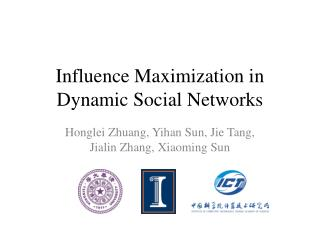Download PresentationInfluence Maximization in Dynamic Social Networks

# Influence Maximization in Dynamic Social Networks

Download Presentation## Influence Maximization in Dynamic Social Networks

- - - - - - - - - - - - - - - - - - - - - - - - - - - E N D - - - - - - - - - - - - - - - - - - - - - - - - - - -
##### Presentation Transcript

1. Influence Maximization in Dynamic Social Networks HongleiZhuang, Yihan Sun, Jie Tang, Jialin Zhang, Xiaoming Sun

2. Influence Maximization Probability of influence B How to find influential users to help promote a new product? A 0.8 C ? 0.1 0.5 0.4 0.6 0.1 0.6 Marketer Alice D E F 0.1 Find K nodes (users) in a social network that could maximize the spread of influence (Domingos, 01; Richardson, 02; Kempe, 03)

3. Influence Maximization • Influence model • Initially all users are considered inactive • Then the chosen users are activated, who may further influence their friends to be active as well • Models • Linear Threshold model • Independent Cascading model  D. Kempe, J. Kleinberg, and E. Tardos. Maximizing the spread of influence through a social network. KDD’03, pages 137–146, 2003.

4. Approximate Solution 00 The problem is solved by optimizing a monotonic submodular function • NP-hard  • Linear Threshold Model • Independent Cascading Model • KempeProve that approximation algorithms can guarantee that the influence spread is within(1-1/e) of the optimal influence spread. • Verify that the two models can outperform the traditional heuristics • Recent research focuses on the efficiency improvement •  accelerate the influence procedure by up to 700 times • It is still challenging to extend these methods to large data sets  D. Kempe, J. Kleinberg, and E. Tardos. Maximizing the spread of influence through a social network. KDD’03, pages 137–146, 2003.  J. Leskovec, A. Krause, C. Guestrin, C. Faloutsos, J. VanBriesen, and N. Glance. Cost-effective outbreak detection in networks. KDD’07, pages 420–429, 2007.

5. Influence Maximization in Dynamic Networks Evolve Probe Original edges Added edges Removed edges • About6 millionlinks changed on Weibo network • WeiboAPI limitation: ≤ 450 times/hr

6. Problem • Input: For a dynamic social network {G0,…, Gt}, we have observed G0, but for all t>0, Gt is unknown • Problem: To probe b nodes, observe their neighbors to obtain an observed network from , such that influence maximization on the real network can be approximated by that on the observed network. • Challenge: How to find the influential users, if we only partially observe the update of the social network?

7. Basic Idea • Estimate how likely the neighborhood of a node will change in a dynamic social network • Probe nodes that change a lot • Estimate how much the influence spread can be improved by probing a node • Probe the one maximizes the improvement

8. Methodologies and Results

9. Preliminary Theoretical Analysis • Formal definition of loss • With an specified evolving graph model • At each time stamp an edge is chosen uniformly • and its head will point to a node randomly chosen with probability proportional to the in-degree Max seed set on fully observed network Max seed set on partially observed network

10. Preliminary Theoretical Analysis • Error bound of Random probing strategy • Error bound of Degree weighted probing strategy • In most cases, degree weighted probing strategy performs better than random probing strategy

11. Maximum Gap Probing • Basic Idea • Estimate how much the influence spread can be improved by probing a node • Probe the one which maximizes the improvement • Formally, • For a given tolerance probability • The minimum value that satisfies the following inequality is defined as performance gap Best solution if v is probed Best solution before probing *To simplify problem, define the quality function as the sum of degree in the seed set.

12. Maximum Gap Probing • Assume the degree of a node is a martingale. We can estimate the degree gap of each node by • Considering the node to probe is in/not in the current seed set. • Each time, choose the one with maximum gap to probe Last time when v is probed Defined as zv

13. MaxG Algorithm Finding nodes to probe by maximizing the degree gap Perform the standard greedy algorithm (degree discount heuristics) for influence maximization

14. Experiment Setup • Data sets • Evaluation • Take optimal seed set obtained from partially observed network • Calculate its influence spread on real network

15. Experiment Setup • Comparing methods • Rand, Enum: Uniform probing • Deg, DegRR: Degree-weighted probing • BEST: Suppose network dynamics fully observed • Configurations • Probing budget: • b=1,5 for Synthetic; b=100,500 for Twitter and Coauthor • Seed set size for influence maximization: • k=30 for Synthetic; k=100 for Twitter and Coauthor • Independent Cascade Model, with uniform p=0.01

16. Experimental Results • Average influence spread The large, the best

17. Influence Maximization Results (b=100) Twitter Coauthor

18. Influence Maximization Results (b=500) Twitter Coauthor

19. Conclusions

20. Conclusions • Propose a probing algorithm to partially update a dynamic social network, so as to guarantee the performance of influence maximization in dynamic social networks • Future work include: • Online updating seed set in dynamic social networks • Probing for other applications, e.g. PageRank  B. Bahmani, R. Kumar, M. Mahdian, and E. Upfal. PageRank on an evolving graph. In KDD, pages 24–32, 2012.

21. Thank you • Dec 8, 2013 • Go to… • Motivating Example • Problem Formulation • Approach • Experiments